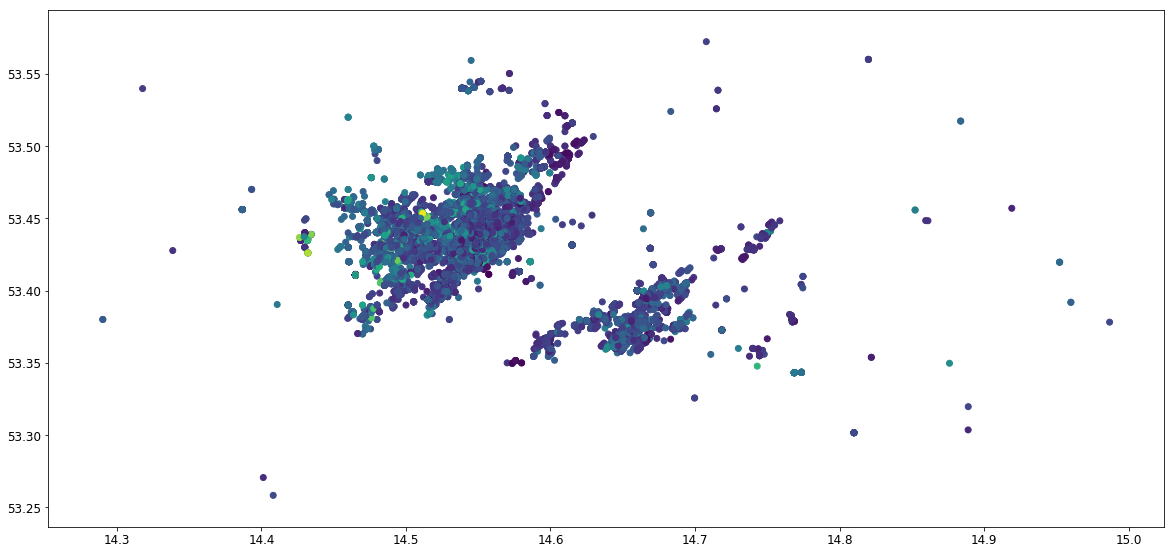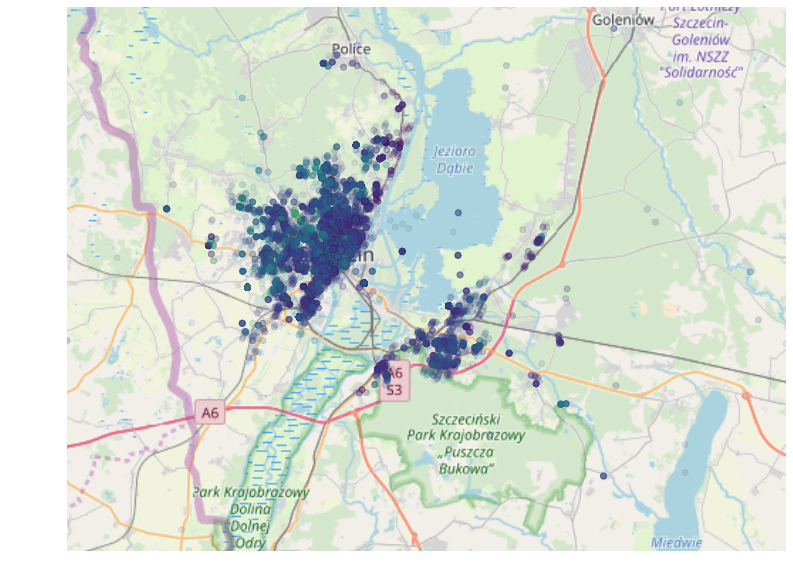# Housing data analysis using Machine Learning - adding geo data

I've started my analysis in part1 from simple data exploration and most basic OLS regression. Then in part 2 I proceeded to include more advanced Machine Learning methods from the sciki-learn library.

In today's part I will move to augmenting my data with geographical characteristics. For that I will explore the OpenStreetMap data for Szczecin and I will merge it with downloaded housing ads. I wanted to see if adding information about proximity to schools, shops, entertainment and other amenities has any influence on housing prices.

Most of the tools using in this chapter I've learned from two books. I already knew some basic SQL syntax, but by studying PostgreSQL: Up and Running I familiarized myself with some peculiarities of PostgreSQL. I tried using MySQL, sqlite and MongoDB, but somehow I grew a liking for the former. Though it might have been the case that was the first time that I learned a relational database systematically from a book, instead of web tutorials. Also whenever I forget a particular topic it is relatively easy for me to find it in a book.

Also I used a lot of GIS recently at work working mostly with QGIS and ArcGIS. I quickly got interested in easy routing calculation using pgRouting. When wanting to go deeper into it I naturally needed to learn PostGIS. For that I have studied it using PostGIS in Action. It was a lifesaver! The book was written brilliantly! I enjoyed going through it both learning more about PostgreSQL and about geo information processing in general. I will implement some of the ideas from the book further in this Notebook.

In the end I will compare different regression models. Finally I will construct a simple Neural Network regression model and compare the results with OLS.

In :
import numpy as np
import psycopg2 as psql
import pandas as pd
import geopandas as gpd

# Matplotlib options
import matplotlib
import matplotlib.pyplot as plt
matplotlib.rcParams['axes.labelsize'] = 14
matplotlib.rcParams['xtick.labelsize'] = 12
matplotlib.rcParams['ytick.labelsize'] = 12
matplotlib.rcParams['figure.figsize'] = (20.0, 10.0)

# Helper function to display PostGIS geometries
def disp_poly(sql, con, geom="geom"):
"""
Display polygon resulting from PostGIS SQL results.
"""
df = gpd.GeoDataFrame.from_postgis(sql, con, geom_col=geom)
if 'id' not in df.columns:
df['id'] = df.index
return df


## Creating distance data¶

After loading basic libraries I will use the IPython SQL magic to connect to PostgreSQL. Also I will create a Psychopg2 connection as well. I will also use pgspecial python module to enable execution of psql commands.

In :
%load_ext sql
%sql postgresql://mateusz@localhost/szczecin_data
con = psql.connect(database="szczecin_data", user="mateusz", host="localhost")

The sql extension is already loaded. To reload it, use:


Before running this exercise I downloaded OpenStreetMap data for Zachodniopomorskie region from Geofabrik.

Next I used osm2pgsql to upload OSM data to PostgreSQL with PostGIS. Resulting tables can be seen as the planet_osm_* tables for points, lines, roads and polygons separately.

In :
%sql \dt

 * postgresql://mateusz@localhost/szczecin_data
8 rows affected.

Out:
Schema Name Type Owner
public dates table mateusz
public planet_osm_line table mateusz
public planet_osm_point table mateusz
public planet_osm_polygon table mateusz
public routing table mateusz
public spatial_ref_sys table mateusz

Remembering from the previous exercise I wanted to filter out some of the observations. I created a view to include only filtered adds, so that I wouldn't need to write the filtering query every time.

DROP VIEW IF EXISTS filtered;
CREATE VIEW filtered AS
SELECT id, price, pow, rooms, floor, data_lat, data_lon, details, description, geom
WHERE
pow < 200 AND
price < 1e6 AND
data_lat > 53.01 AND
data_lat < 53.6 AND
data_lon > 14.01 AND
data_lon < 15 AND
details != '';


### Plotting maps in Python¶

I can now plot the selected adds according to their geographic coordinates. To achieve this I will use a wrapper function around GeoPandas module defined in the beginning.

In :
query = 'SELECT id, pow, price, geom FROM filtered;'
df = disp_poly(query, con)
print(df.shape)

(39373, 4)

Out:
id pow price geom
0 13612 45.94 248000.0 POINT (14.51815711691893 53.38403163098405)
1 14847 90.00 309000.0 POINT (14.47106095 53.38718211)
2 15814 98.60 530000.0 POINT (14.4633004 53.3852974)
3 17486 53.00 289000.0 POINT (14.4755835 53.3887893)
4 17833 100.00 360000.0 POINT (14.4633004 53.3852974)
In :
df.plot(column='price')

Out:
<matplotlib.axes._subplots.AxesSubplot at 0x7ff5a63d6c88>I will next add a map in the background following the GeoPandas documentation.

In :
# Add Helper function for plotting
import contextily as ctx

xmin, xmax, ymin, ymax = ax.axis()
basemap, extent = ctx.bounds2img(xmin, ymin, xmax, ymax, zoom=zoom, url=url)
ax.imshow(basemap, extent=extent, interpolation='bilinear')
# restore original x/y limits
ax.axis((xmin, xmax, ymin, ymax))

# Reproject geo data from WGS84 to Web Mercator
df = df.to_crs(epsg=3857)
# Plot the map with data
ax = df.plot(column='price', alpha=0.1)
minx, miny, maxx, maxy = df.total_bounds
ax.set_xlim(minx, maxx)
ax.set_ylim(miny, maxy)
ax.set_axis_off()That's nice, but there's a lot of going on in the centre. I want to zoom in into a region I defined in a geojson file.

In :
# Read centre